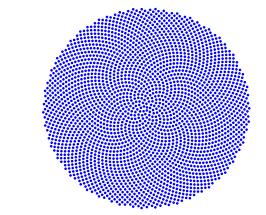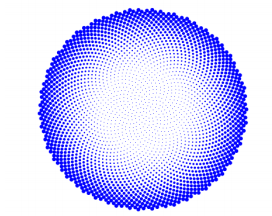# Widen a fibonacci flower using a for cycle

I was wondering in this example that I've used to practice for loops, I needed to change this code in order to change the image that looks like this, some basic golden angle plot:to this example``````    phi = (sqrt(5)+1)/2;% Número áureo %Golden Number
golden_angle = 2*pi/phi;
max_angle = 10000;
theta = 1:golden_angle:max_angle;% ángulo %Angle
[x,y] = pol2cart(theta,r);% coordenadas polares a cartesianas %Polar to cartesian
figure
plot(x,y,'.','MarkerSize',10);axis off;
``````

I know that the number next to 'MarkerSize' wides the flower petals, but I tried changing theta values in the array but with no particular result, only I’ve changed color but not the size. I also tried making these a for cycle, that creates circles as the golden flower petals, I think, but don't know how to implement it. But how to make them more and more small at the iteration goes?, and I think total number of petals is 2575.

``````for i = 1:10

%plot(x,y,'.',x(i),y(i),'.','MarkerSize',10*abs(y(i)),'MarkerFaceColor','g')
``````